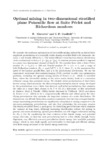## Optimal mixing in two-dimensional stratified plane Poiseuille flow at finite Peclet and Richardson numbers

##### Authors
Caulfield, CP
Marcotte, Florence
##### DOIArticle
##### Abstract

We consider the nonlinear optimisation of irreversible mixing induced by an initial finite amplitude perturbation of a statically stable density-stratified fluid with kinematic viscosity ν and density diffusivity κ. The initial diffusive error function density distribution varies continuously so that ρ∈[ρ¯−(1/2)ρ0,ρ¯+(1/2)ρ0]. A constant pressure gradient is imposed in a plane two-dimensional channel of depth 2h. We consider flows with a finite P'eclet number Pe=Umh/κ=500 and Prandtl number Pr=ν/κ=1, and a range of bulk Richardson numbers Rib=gρ0h/(ρ¯U2)∈[0,1] where Um is the maximum flow speed of the laminar parallel flow, and g is the gravitational acceleration. We use the constrained variational direct-adjoint-looping (DAL) method to solve two optimization problems, extending the optimal mixing results of Foures, Caulfield & Schmid (2014) to stratified flows, where the irreversible mixing of the active scalar density leads to a conversion of kinetic energy into potential energy. We identify initial perturbations of fixed finite kinetic energy which maximize the time-averaged perturbation kinetic energy developed by the perturbations over a finite time interval, and initial perturbations that minimise the value (at a target time, chosen to be T=10) of a mix-norm' as first introduced by Mathew, Mezic \& Petzold (2005), further discussed by Thi eault (2012) and shown by Foures et al. (2014) to be a computationally efficient and robust proxy for identifying perturbations that minimise the long-time variance of a scalar distribution. We demonstrate, for all bulk Richardson numbers considered, that the time-averaged-kinetic-energy-maximising perturbations are significantly suboptimal at mixing compared to the mix-norm-minimising perturbations, and also that minimising the mix-norm remains (for density-stratified flows) a good proxy for identifying perturbations which minimise the variance at long times. Although increasing stratification reduces the mixing in general, mix-norm-minimising optimal perturbations can still trigger substantial mixing for $Ri_b \lesssim 0.3$. By considering the time evolution of the kinetic energy and potential energy reservoirs, we find that such perturbations lead to a flow which, through Taylor dispersion, very effectively converts perturbation kinetic energy into available potential energy', which in turn leads rapidly and irreversibly to thorough and efficient mixing, with little energy returned to the kinetic energy reservoirs.

2018-10-25
2018-08-23
2018-07-12
##### Keywords
mixing enhancement, stratified flows, variational methods
##### Journal Title
Journal of Fluid Mechanics
1469-7645
1469-7645
853
##### Publisher
Cambridge University Press